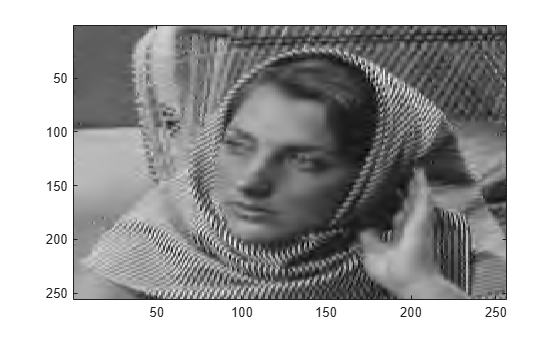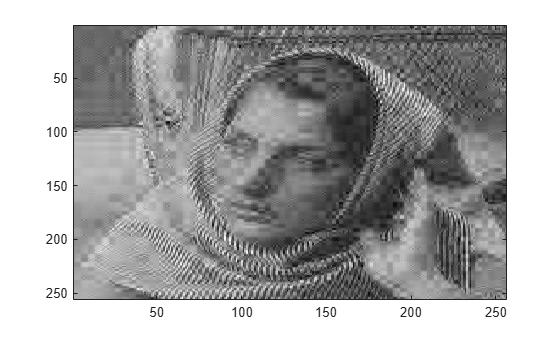# Image Reconstruction with Biorthogonal Wavelets

This example shows how applying the order biorthogonal wavelet filters can affect image reconstruction.

Generate the analysis and synthesis filters for the `bior3.5` wavelet. Load in and display an image.

```[LoD,HiD,LoR,HiR] = wfilters('bior3.5'); load woman imagesc(X) colormap gray```The analysis filters, `LoD` and `HiD`, have 5 vanishing moments. The synthesis filters, `LoR` and `HiR`, have 3 vanishing moments. Do a five-level wavelet decomposition of the image using the analysis filters.

`[c1,s1] = wavedec2(X,5,LoD,HiD);`

Find the threshold that keeps only those wavelet coefficients with magnitudes in the top 10 percent. Use the threshold to set the bottom 90 percent of coefficients to 0.

```frac = 0.1; c1sort = sort(abs(c1),'desc'); num = numel(c1); thr = c1sort(floor(num*frac)); c1new = c1.*(abs(c1)>=thr);```

Reconstruct the image using the synthesis filters and the thresholded coefficients. Display the reconstruction.

```X1 = waverec2(c1new,s1,LoR,HiR); figure imagesc(X1) colormap gray```Do a five-level wavelet decomposition of the image using the synthesis filters.

`[c2,s2] = wavedec2(X,5,LoR,HiR);`

Find the threshold that keeps only those wavelet coefficients with magnitudes in the top 10 percent. Use the threshold to set the bottom 90 percent of coefficients to 0

```frac = 0.1; c2sort = sort(abs(c2),'desc'); num = numel(c2sort); thr = c2sort(floor(num*frac)); c2new = c2.*(abs(c2)>=thr);```

Reconstruct the image using the synthesis filters and the thresholded coefficients. Display the reconstruction. Decomposing with a filter that has 3 vanishing moments and reconstructing with a filter that has 5 vanishing moments results in poor reconstruction.

```X2 = waverec2(c2new,s2,LoD,HiD); figure imagesc(X2) colormap gray```